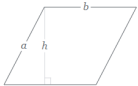Q:

# Write an algorithm, pseudocode and draw a flowchart of finding the area of parallelogram

Write an algorithm, pseudocode and draw a flowchart of finding the area of parallelogram

Area= B*H where B is the base, H is the height